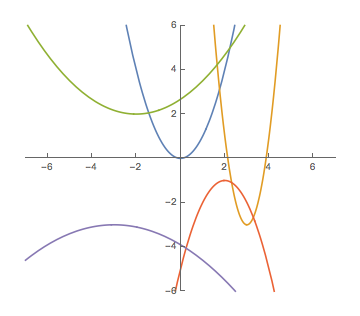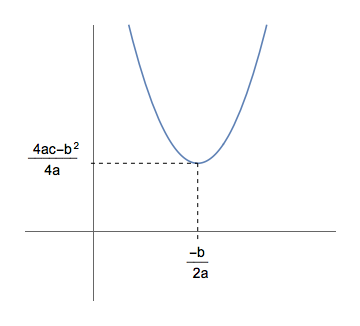## Parabolas

Let us now consider the graphs of polynomial functions of degree two -- that is to say, quadratic functions of the form $$f(x)=ax^2 + bx + c$$ for some constants $a$, $b$, and $c$.

Plotting a bunch of points for some randomly chosen quadratic functions reveals some interesting properties, as seen below. All of the quadratic functions, when plotted, appear to take on a "U"-like shape -- although sometimes this "U" is upside down. As a result, the quadratic functions appear to have either a maximum or minimum value, depending on whether the "U" is upside-down or right-side-up, respectively. The graph of a quadratic function (which we call a parabola) seems to sometimes be "wide", sometimes "narrow", and still other times something between those two extremes. They sometimes cross the $x$-axis producing two $x$-intercepts. Other times, the only touch the $x$-axis once, or not at all. They always have a $y$-intercept (granted, our graph has to be big enough to show it).Let's see if we can't consider each of these ideas a bit more carefully...

First, with regards to deducing the shape of the graph of this function from it's equation -- note, things would probably be easier to analyze if we could rewrite the equation for the function using only a single occurrence of $x$. Recall the trick employed to the same end when solving general quadratic equations -- namely, "completing the square"...

$$\begin{array}{rcl} f(x) &=& ax^2 + bx + c\\\\ &=& a(x^2 + \frac{b}{a}x\, + \,?\,) + c\\\\ &=& a(x^2 + \frac{b}{a}x + \frac{b^2}{4a^2}) + c - \frac{b^2}{4a}\\\\ &=& a(x + \frac{b}{2a})^2 + \frac{4ac - b^2}{4a} \end{array}$$

Now, let us consider the factor of the first term seen in the last line above: $(x + \frac{b}{2a})^2$. This term is certainly positive, by virtue of being a square -- and thus equivalent to

$$\left| x + \frac{b}{2a} \right|^2 = \left| x - (-\frac{b}{2a}) \right|^2$$

This might initially seem to be a strange way to rewrite $f(x)$, but remember that $|p-q|$ gives the distance between $p$ and $q$ on the real number line. So the expression above is the square of the distance between $x$ and $-\frac{b}{a}$. Armed with this interpretation, we can see that as $x$ gets farther and farther from $-\frac{b}{2a}$, the expression above gets larger and larger in magnitude.

Also note that in finding the height of the graph of $f(x)$ at some particular $x$, $$\begin{array}{rcl} f(x) &=& a(x + \frac{b}{2a})^2 + \frac{4ac - b^2}{4a}\\\\ &=& a \left| x - (-\frac{b}{2a}) \right|^2 + \frac{4ac - b^2}{4a} \end{array}$$ we see that the amount added to $\frac{4ac - b^2}{4a}$ depends only on the distance between $x$ and $-\frac{b}{a}$, and not whether $x$ is to the right or left of this value.

This confirms our suspicion that parabolas are symmetric. In fact, we can say even more -- the axis of symmetry of a parabola described by $f(x)=ax^2+bx+c$ is the vertical line $x=-\frac{b}{a}$.

Now consider the impact of the sign of $a$. When $a$ is positive, for any $x \ne -\frac{b}{2a}$, we are adding a positive value to $\frac{4ac - b^2}{4a}$ -- and one that grows as $x$ gets farther from $-\frac{b}{2a}$. Consequently, if $a > 0$, the function $f(x)$ has a minimum value of $\frac{4ac - b^2}{4a}$ at $x = -\frac{b}{2a}$, and the graph is basically "U"-shaped -- where the direction of opening is "up".Alternatively, when $a$ is negative, then for any $x \ne -\frac{b}{2a}$, we are always subtracting some positive value from $\frac{4ac - b^2}{4a}$ -- again, one that grows in magnitude as $x$ gets farther from $-\frac{b}{2a}$. So, if $a < 0$, the function $f(x)$ has a maximum value of $\frac{4ac - b^2}{4a}$ at $x = -\frac{b}{2a}$, and the graph is also shaped like a "U", only upside-down (i.e., the direction of opening is "down").

The reader should reflect on the impact of the magnitude of $a$ as well. Upon doing so, one can quickly conclude that the greater the magnitude of $a$, the more steeply the curve ascends (or descends, as appropriate). Consequently, large magnitudes of $a$ correspond to "narrow" parabolas, while small magnitudes of $a$ correspond to "wide" parabolas.

As a matter of verbiage, the special point $(-\frac{b}{2a}, \frac{4ac - b^2}{4a})$ where either the maximum or minimum occurs we refer to as the vertex of the parabola.

It's worth noting that if we are given the quadratic function $f(x) = ax^2 + bx + c$ and we complete the square to rewrite the function in the form $f(x)=a(x-h)^2 + k$ as we did above, then $h = -\frac{b}{2a}$ and $k = \frac{4ac - b^2}{4a}$.

Consequently, if someone has already done some of the work for us and given us a quadratic function in the form $f(x)=a(x-h)^2 + k$, then the vertex must be given by $(h,k)$.

Finally, with regards to the $x$-intercepts, recall that points on the $x$-axis have a $y$-coordinate of zero. As such, we can find all $x$ intercepts by solving

$$ax^2 + bx + c = 0$$

Being a quadratic equation, we know the solution to the above takes the form

$$x = \frac{-b \pm \sqrt{b^2 - 4ac}}{2a}$$

This results in $0$, $1$, or $2$ solutions for $x$, depending on the value of the discriminant $b^2-4ac$.

As an immediate consequence -- there are two $x$-intercepts when the discriminant is positive; one $x$-intercept when the discriminant is zero; and no $x$-intercepts when the discriminant is negative.